Next: 2.4 Demands for the Up: 2. Grid Types Previous: 2.2 Tetrahedral Grids

# 2.3 Grid Requirements

To obtain quality criteria for the grids, the differential equations of the models must be taken into account. For the solution of the frequently required Laplace equation and the diffusion equation, an additionally required grid-condition must be formulated. It will be helpful to illustrate it by an example. When solving the electrostatic potential distribution inside a capacitor it is physically plausible that, if the voltages on the contacts lie in the range of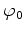to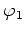, all potential values inside the capacitor also must lie within the same range.

Mathematically, this is known as the maximum principle . In its simplest form it states that both the maximum and the minimum values occur on the boundary or at initial time. Since the numerical solution must be a good approximation of the exact solution and unphysical solutions must be prevented, this maximum principle has to be satisfied also by the numerical method. For the diffusion equation, this principle implies that unphysical negative concentrations may not appear in the solution (as negative concentrations do not appear in the initial condition). This principle implies also another physical interpretation, which is known as the Positive Transmissibility Condition. It states, that the physical flow has to be directed from higher to lower concentrations.

Within the discretization methods, which are in particular the Box Integration, as well as the Finite Element discretization with a standard Galerkin weighted residual approach, the Laplace equation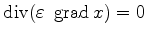(2.1)

and the diffusion equation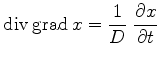(2.2)

result in the same type of equation system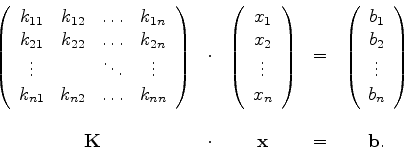(2.3)

The maximum principle is satisfied if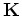becomes an M-matrix .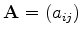is an M-matrix , if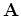is a real and nonsingular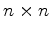matrix, where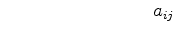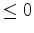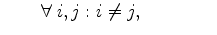(2.4)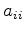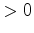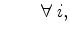(2.5)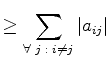(2.6)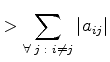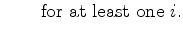(2.7)

The entries of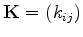differ in the composition of left-hand and right-hand terms of equation (2.1), or (2.2) with backward Euler time discretization .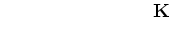for (2.1) and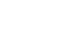(2.8)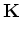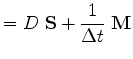for (2.2). (2.9)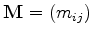denotes the mass matrix, which arises from the time variant right-hand side,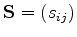is the stiffness matrix, which depends only on the left-hand side of equation (2.1) or (2.2). For this composition we know thatis an M-matrix ifis a positive definite diagonal matrix and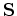also is an M-matrix. For Box Integration (refer Section 3.2), the positive definite diagonal characteristic ofis satisfied. For Finite Elements, mass matrix lumping has to be performed to achieve a positive definite diagonal matrix. Sinceonly depends on the mesh, this condition translates to a grid constraint. Off-diagonal elements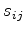ofmust not be positive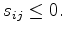(2.10)

This constraint also fulfills the Positive Transmissibility Condition .

Consequentially the requirements for Box Integration and Finite Elements follow.Next: 2.4 Demands for the Up: 2. Grid Types Previous: 2.2 Tetrahedral Grids

J. Cervenka: Three-Dimensional Mesh Generation for Device and Process Simulation